### Home > CCA > Chapter Ch8 > Lesson 8.2.2 > Problem8-71

8-71.
1. For each equation below, solve for x. Homework Help ✎

1. (x − 2)(x + 8) = 0

2. (3x − 9)(x − 1) = 0

3. (x + 10)(2x − 5) = 0

4. (x − 7)2 = 0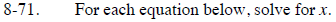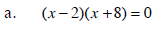In order for a product to be 0, one of the factors must be equal to 0.

x − 2 = 0 or x + 8 = 0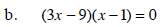Follow the steps in part (a).

x = 3 or 1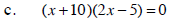Follow the steps in part (b).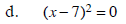( x − 7 )2 = ( x − 7 )( x − 7 )

Follow the steps in part (a).